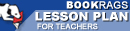Lesson Plans

# Journey Through Genius: The Great Theorems of Mathematics Quiz | Eight Week Quiz D

This set of Lesson Plans consists of approximately 142 pages of tests, essay questions, lessons, and other teaching materials.
 View a FREE sampleName: _________________________ Period: ___________________

This quiz consists of 5 multiple choice and 5 short answer questions through Cardano and the Solution of the Cubic.

## Multiple Choice Questions

1. Which words best describe how solid proofs were developed in Elements?
(a) Axiomatic framework.
(b) Inverted scaffold.
(c) Programmed order.
(d) Simple arguments.

2. What did Euclid do in his 48th proposition?
(a) Euclid demonstrated the faults of the Pythagorean Theorem.
(b) Euclid demonstrated how to use the Pythagorean Theorem.
(c) Euclid proved the converse of the Pythagorean Theorem.
(d) Euclid proved the Pythagorean Theorem.

3. Which of the following is an example of a postulate that must be accepted in Elements?
(a) It is possible to draw a straight line between an infinite number of points.
(b) It is possible to draw an arc with any three points.
(c) It is possible to draw a circle that contains no lines.
(d) It is possible to connect any two points with a line and make a circle.

4. What is true about prime numbers?
(a) Prime numbers can never be an odd number.
(b) Prime numbers are not divisible by other numbers.
(c) That for every group of prime numbers, there exists at least one more prime.
(d) Prime numbers can not exist in a finite series.

5. Besides being a mathematician, what else other work was Archimedes famous for?
(a) Doctor and writer,
(b) Politician.
(c) Inventor and scientist.
(d) Artist and musician.

1. Which of the following was NOT defined by Euclid?

2. Which is a geometric concept that humans have been aware of since the dawn of agriculture?

3. What does the Pythagorean Theorem state?

4. Which of the following was NOT one of Gauss' discoveries?

5. What was the title of Cardano's book which contained the solution to the cubic?

 This section contains 378 words (approx. 2 pages at 300 words per page) View a FREE sample